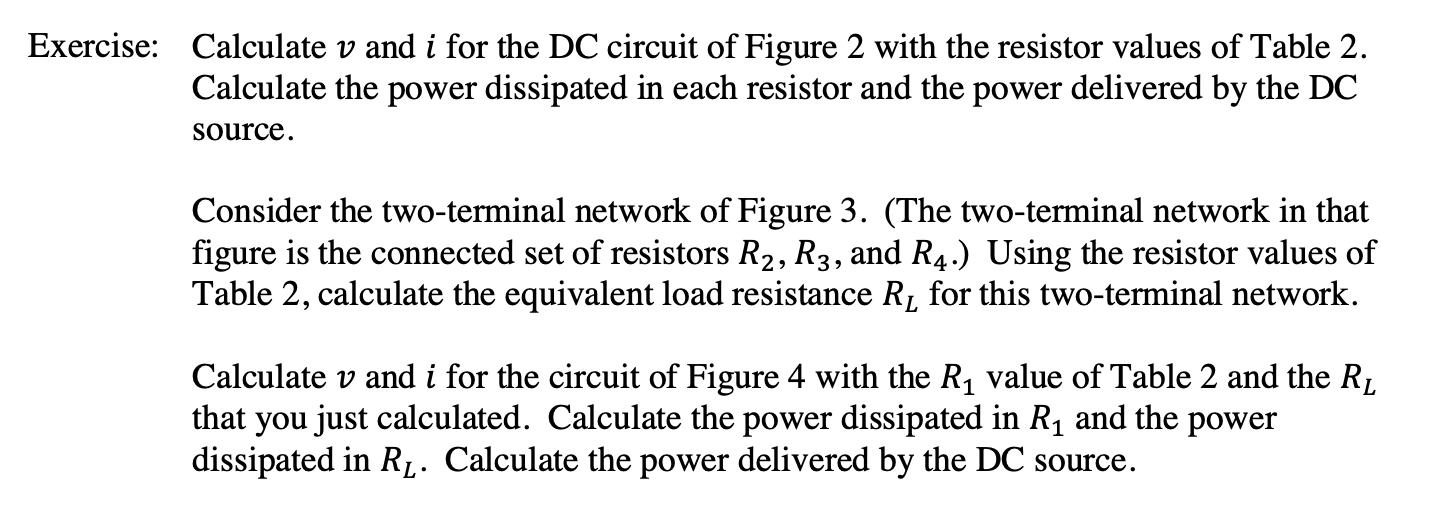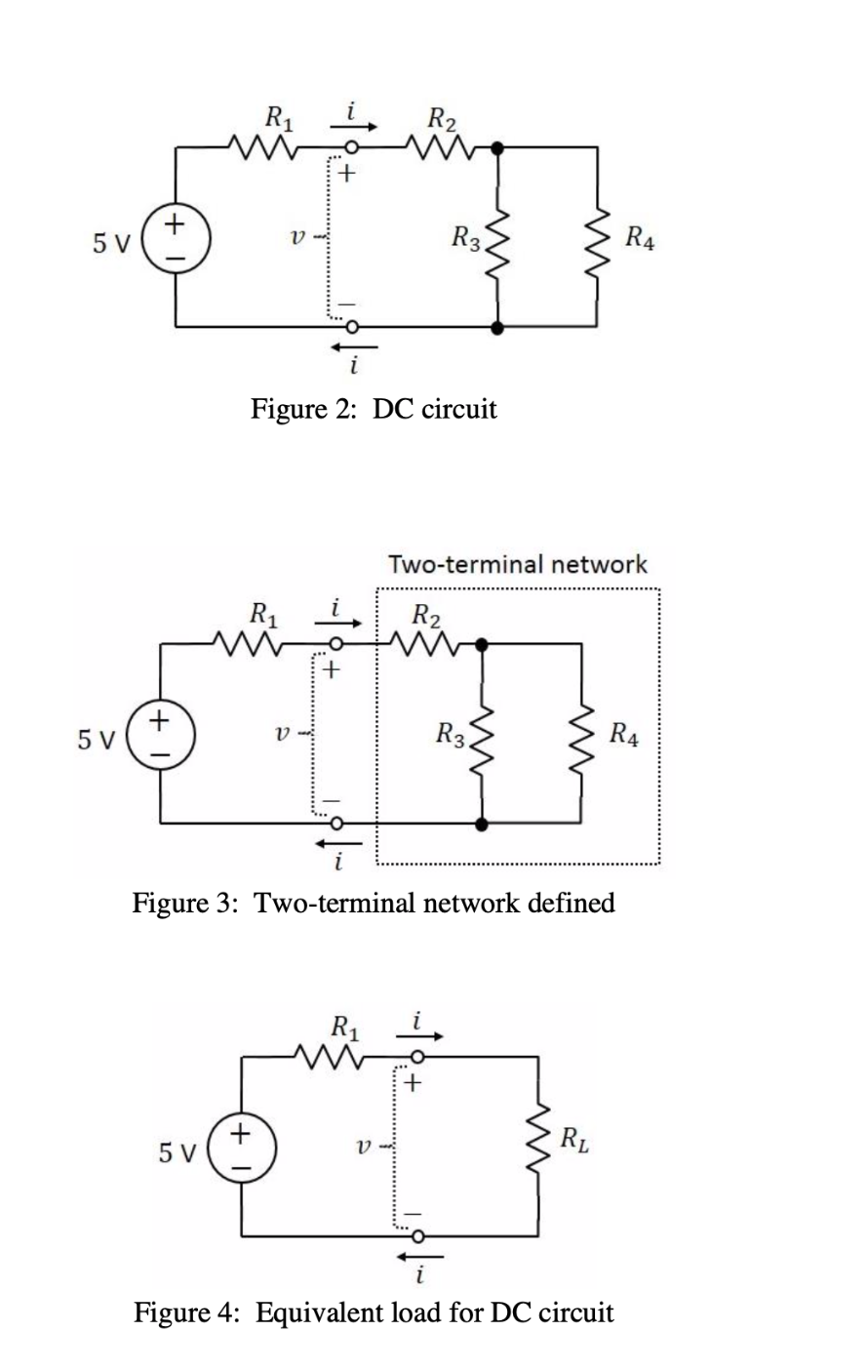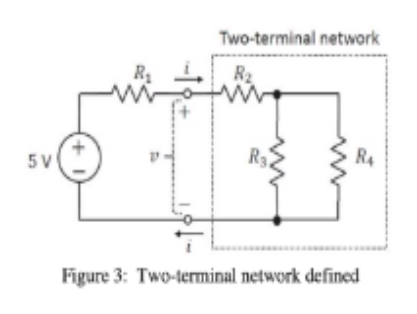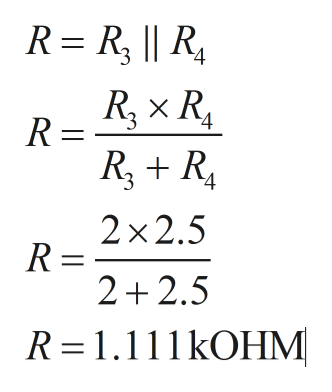Calculate v and i for the DC circuit of Figure 2 with the resistor values of Table 2Calculate the power dissipated in each resistor and the power delivered by the DCExercise:sourceConsider the two-terminal network of Figure 3. (The two-terminal network in thatfigure is the connected set of resistors R2, R3, and R4.) Using the resistor values ofTable 2, calculate the equivalent load resistance Rj for this two-terminal networkCalculate v and i for the circuit of Figure 4 with the R1 value of Table 2 and the Rjthat you just calculated. Calculate the power dissipated in R1 and the powerdissipated in Rf. Calculate the power delivered by the DC source R2R3R4V5 VFigure 2: DC circuitTwo-terminal networkR2R1+5 VR3R4VFigure 3: Two-terminal network definedR1++5 VVFigure 4: Equivalent load for DC circuitw

Question
 Resistor Value R1 3kOHM R2 1kOHM R3 2.5kOHM R4 2.0kOHM
Table 2

Please answer second question (the one in the middle)help_outlineImage TranscriptioncloseCalculate v and i for the DC circuit of Figure 2 with the resistor values of Table 2 Calculate the power dissipated in each resistor and the power delivered by the DC Exercise: source Consider the two-terminal network of Figure 3. (The two-terminal network in that figure is the connected set of resistors R2, R3, and R4.) Using the resistor values of Table 2, calculate the equivalent load resistance Rj for this two-terminal network Calculate v and i for the circuit of Figure 4 with the R1 value of Table 2 and the Rj that you just calculated. Calculate the power dissipated in R1 and the power dissipated in Rf. Calculate the power delivered by the DC source fullscreenhelp_outlineImage TranscriptioncloseR2 R3 R4 V 5 V Figure 2: DC circuit Two-terminal network R2 R1 + 5 V R3 R4 V Figure 3: Two-terminal network defined R1 + + 5 V V Figure 4: Equivalent load for DC circuit w fullscreen
Step 1

Considering the circuit number two,help_outlineImage TranscriptioncloseTwo-terminal network R2 5 V Figure 3: Two-terminal network defined fullscreen
Step 2

Resistance R3 and R4 are in parallel their equivalent resistance is given by R,help_outlineImage TranscriptioncloseR R, || R R R RR 2x2.5 R = 2 2.5 R 1.111kOHM fullscreen
Step 3

The simplified circuit is repr...

Want to see the full answer?

See Solution

Want to see this answer and more?

Our solutions are written by experts, many with advanced degrees, and available 24/7

See Solution
Tagged in

Electrical Engineering